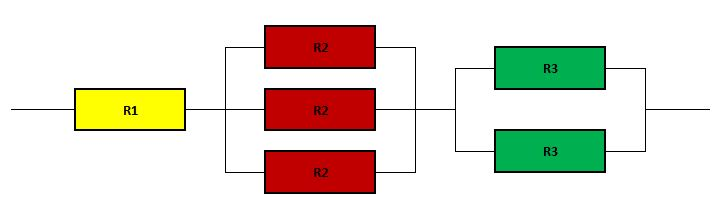# Problem 49850. Simple Circuit of Resistors

The configuration of a group of resistors is described in a matrix with two rows. The first row provides the information regarding the number of resistors at each junction and the second row provides the resistance of each detector at each junction. Consider the following configuration:The circuit_def (i.e., the matrix defining the problem) for this configuration is [1 3 2; R1 R2 R3]. Find the resultant resistance for any given configuration.

### Solution Stats

68.75% Correct | 31.25% Incorrect
Last Solution submitted on Oct 11, 2023

### Community Treasure Hunt

Find the treasures in MATLAB Central and discover how the community can help you!

Start Hunting!